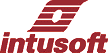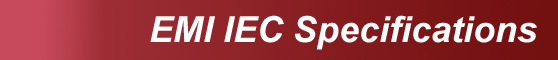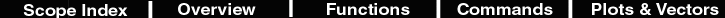Usage Note: To receive data, first input the line current and probe. Press F for a Fourier transform of current. The result in IntuScope5 is a bar graph with data that is presented as triangles. That data is plotted against the specification limits for the particular equipment class. You must have the current vector for this to run.

Class A Equipment
Description IEC 61000 Electromagnetic Interference Specifications (Limits for Harmonic Current Emissions) for Class A Equipment. This specifies limits of harmonic components of the input current which may be produced by balanced three-phase equipment; household appliances, excluding equipment identified as Class D; tools, excluding portable tools; dimmers for incandescent lamps; and audio equipment.

Table 1 - Limits for Class A equipment

Harmonic order

n

Maximum permissible
harmonic current
A
Odd harmonics
 3 2,30 5 1,14 7 0,77 9 0,40 11 0,33 13 0,21 15 <= n <= 39 0,15 15/n
Even harmonics
 2 1,08 4 0,43 6 0,30 8 <= n <= 40 0,23 8/n
Script *Class A Spec current plot must be 2048 points in frequency (default FFT)
assertvalid current frequency
if length(current) <> 2048
printtext "Incorrect lewngth for frequency"
else
numHarmonics = 20
ilimit = 0*vector(numHarmonics)
frequency = (freq+j(0))*vector(numHarmonics)
setunits frequency hertz
ilimit = 2.3
ilimit = 1.14
ilimit = .77
ilimit = .4
ilimit = .33
ilimit = .21
n=15
while n < numHarmonics
ilimit[n] = 2.25/n
n = n + 2
end
ilimit = 1.08
ilimit = .43
ilimit = .3
n=8
while n < numHarmonics
ilimit[n] = 1.84/n
n=n+2
end
Imax = 4
settracecolor rgb 0 64 0
settracestyle triupdot wide
setunits frequency hertz
setunits ilimit amps
setautoscale all
plotref ilimit frequency
setxlimits 0 freq*numHarmonics
settracecolor rgb 128 0 0
settracestyle bdiagbar wide
end

Class B Equipment
 Description IEC 61000 Electromagnetic Interference Specifications (Limits for Harmonic Current Emissions) for Class B Equipment. This specifies limits of harmonic components of the input current which may be produced by portable tools and arc welding equipment, which is not professional equipment. Script *Class B Spec current plot must be 2048 points in frequency (default FFT) assertvalid current frequency if length(current) <> 2048 printtext "Incorrect lewngth for frequency" else askvalues freq "Frequency" numHarmonics = 20 ilimit = 0*vector(numHarmonics) frequencyspec = (freq+j(0))*vector(numHarmonics) setunits frequencyspec hertz ilimit = 3.45 ilimit = 1.71 ilimit = 1.155 ilimit = .6 ilimit = .495 ilimit = .315 n=15 while n < numHarmonics ilimit[n] = 3.375/n n = n + 2 end ilimit = 1.62 ilimit = .645 ilimit = .45 n=8 while n < numHarmonics ilimit[n] = 2.76/n n=n+2 end Imax = 4 settracecolor rgb 0 64 0 settracestyle triupdot wide setunits frequencyspec hertz setunits ilimit amps setautoscale all plotref ilimit frequencyspec setxlimits 0 freq*numHarmonics settracecolor rgb 128 0 0 settracestyle bdiagbar wide end

Class C Equipment
Description IEC 61000 Electromagnetic Interference Specifications (Limits for Harmonic Current Emissions) for Class C Equipment. This specifies limits of harmonic components of the input current which may be produced by lighting equipment.

Table 2 - Limits for Class C equipment

Harmonic order

n

Maximum permissible harmonic current
expressed as a percentage of the input
current at the fundamental frequency
%
 2 2 3 30 * landa+ 5 10 7 7 9 5 11 <= n <= 39 (odd harmonics only) 3
+ landa is the circuit power factor
Script

*Class C Spec current plot must be 2048 points in frequency (default FFT)
assertvalid current frequency
if length(current) <> 2048
printtext "Incorrect lewngth for frequency"
else
askvalues freq "Frequency" pf "Power Factor"
numHarmonics = 12
tmp = mag(current)
setcursor 0 0
setcursor 1 mag(frequency)
inCurrent = sqrt(2048*rmspts(tmp )^2)
print inCurrent
ilimit = 0*vector(numHarmonics)
frequencyspec = (freq+j(0))*vector(numHarmonics)
setunits frequencyspec hertz
ilimit = 0.30*pf*inCurrent
ilimit = 0.1*inCurrent
ilimit = 0.07*inCurrent
ilimit = 0.05*inCurrent
ilimit =0.03*inCurrent
ilimit = 0.02*inCurrent
settracecolor rgb 0 64 0
settracestyle triupdot wide
setunits ilimit amps
setautoscale all
plotref ilimit frequencyspec
setxlimits 0 freq*numHarmonics
settracecolor rgb 128 0 0
settracestyle bdiagbar wide
end

Class D Equipment
Description IEC 61000 Electromagnetic Interference Specifications (Limits for Harmonic Current Emissions) for Class D Equipment. This specifies limits of harmonic components of the input current which may be produced by personal computers and personal computer monitors; television receivers, both with a specified power of less than or equal to 600W.
Table 3- Limits for Class D equipment

Harmonic order

n

Maximum permissible
harmonic current
per watt
mA/W

Maximum permissible
harmonic current

A

 3 3,4 2,30 5 1,9 1,14 7 1,0 0,77 9 0,5 0,40 11 0,35 0,33 14 <= n <= 39 (odd harmonics only) 3,85/n See table 1
Script *Class D Spec current plot must be 2048 points in frequency (default FFT)
assertvalid current frequency
if length(current) <> 2048
printtext "Incorrect lewngth for frequency"
else
numHarmonics = 20
print pwr
print freq
ilimit = 0*vector(numHarmonics)
frequency = (freq+j(0))*vector(numHarmonics)
if pwr > 75
dP = pwr - 75
if pwr < 400
ilimit = .275 + 3.4m*dP
ilimit = .175+1.3m*dP
ilimit = .125+1m*dP
ilimit = .100+.4m*dP
n=11
while n < numHarmonics
ilimit[n] = .5/n+3.3m*dP/n
n = n + 2
end
ilimit = .1+.4m*dP
ilimit = .05 + .2m*dP
n=6
while n < numHarmonics
ilimit[n] = .05
n = n + 2
end
Imax = 1
else
ilimit = 1.38
ilimit = .6
ilimit = .45
ilimit = .23
n=11
while n < numHarmonics
ilimit[n] = 1.62/n
n = n + 2
end
ilimit = .23
ilimit = .12
n = 6
while n < numHarmonics
ilimit[n] = .05
n = n + 2
end
Imax = 2
end
else
ilimit = .275
ilimit = .175
ilimit = .125
ilimit = .100
n=11
while n < numHarmonics
ilimit[n] = .55/n
n = n + 2
end
ilimit = .1
ilimit = .05
n = 6
while n < numHarmonics
ilimit[n] = .025
n = n + 2
end
Imax = .5
end
settracecolor rgb 0 64 0
settracestyle triupdot wide
setunits frequency hertz
setunits ilimit amps
setautoscale all
plotref ilimit frequency
setxlimits 0 freq*numHarmonics
settracecolor rgb 128 0 0
settracestyle bdiagbar wide
end